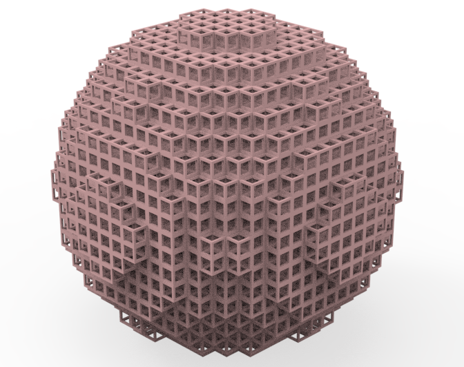# # 3D optical Luneburg lens

Luneburg lens is a prototypical gradient index (GRIN) optical component. A classical Luneburg lens is a spherical lens with a spatially varying refractive index profile following , where is the radial distance, is the radius of the lens, and is the refractive index of the ambient environment. Plane wave incident on a Luneburg lens will be focused to a point on the surface of the lens. Compared to a usual refractive lens, Luneburg lens is abberation-free and coma-free, which enables a wide range of applications in modern optical systems.

However, it is practically difficult to construct such a lens due to the required gradient index distribution. In the microwave regime, high-gain antennas based on a Luneburg lens design can be achieved by, for example, using concentric ceremics shells with different densities. In the optical frequencies, such an approach is generally not applicable.

In this notebook, we demonstrate the numerical simulation of a practical 3D optical Luneburg lens. The structure consists of a large number of subwavelength unit cells. Using an effective medium approach, each unit cell can be approximated by a local effective index, which can be tuned by the filling fraction of the dielectric polymer in the unit cell. By varying the filling fraction of each unit cell such that the local effective index follows , a Luneburg lens is constructed. This design is adapted from Zhao, Y. Y. et al. Three-dimensional Luneburg lens at optical frequencies. Laser Photonics Rev. 10, 665–672 (2016) (opens new window). In the simulation, a linearly polarized plane wave is launched towards the Luneburg lens. Through the visualization of the field distribution, the focusing capability of the lens can be assessed. We also compare the practical Luneburg lens design with the idealized case, which is simulated using CustomMedium (opens new window). The comparison result shows the practical Luneburg lens design is very optimal.